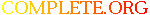Complete.Org: Mailing Lists: Archives: freeciv-ai: August 2004: [freeciv-ai] Re: (PR#9623) Re: terrain improvers (fwd)# [freeciv-ai] Re: (PR#9623) Re: terrain improvers (fwd)

[Top] [All Lists]

 To: undisclosed-recipients: ; Subject: [freeciv-ai] Re: (PR#9623) Re: terrain improvers (fwd) From: "Gregory Berkolaiko" Date: Fri, 6 Aug 2004 10:43:46 -0700 Reply-to: rt@xxxxxxxxxxx

```<URL: http://rt.freeciv.org/Ticket/Display.html?id=9623 >

Thoughts on terrain improvers:

this is a try to give a quasi-scientific basis to evaluating terrain
improvements.

Assumption: somebody else supplies resource weights, workers don't have to

tile_value is basically resources we get from a tile multiplied by their
weights.

benefit = tile_value_after - tile_value_before
if the tile is worked and
= tile_value_after - worst_worked_tile_value
if the tile is not worked

we assume that this benefit is "paid" to us every turn starting from the
moment the improvement is built, which is

d = move_time + time_to_improve
(d for delay)

q is the "inflation multiplier", currently (MORT-1)/MORT, used in
amortization

the total benefit (till the end of time) that we will get from an
improvement is
total_benefit = benefit * (q^d + q^(d+1) + ...)
= benefit * q^d / (1-q)
= benefit * q^d * MORT

mantaining workers also cost so

cost = value of resources spent in one turn on mantaining the worker
(in default rules = 1 shield)

we will be paying them for d turns, so
total_cost = cost * (1 + q + q^2 + ... q^(d-1))
= cost * (1-q^d) / (1-q)
= cost * (1-q^d) * MORT

total_benefit - total_cost would give you want of making the improvement.

This is all sweet theory but (1) it doesn't quite work with the present
weights and (2) might not work with any weights

To give examples:
building irrigation on an adjacent grassland = 261
building mine on an unworked hill (assuming we will switch there from a
unimproved grassland) = 328

I would build road first, then (maybe) irrigation and then a mine.  It can
be argued that it's a matter of taste.

But the biggest problem I see is actually making sure that a switch to the

Ideally, the benefit would be the increase of CMA score once the
improvement is built.  But is it possible to calculate in finite time?

G.

P.S. Evaluating the want to build a worker is even harder, we have to
somehow take into account how much work is available.

```